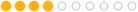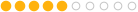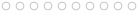### Houdini to Different Software Axis Conversion Lookup Table

3350   2   3スタッフ95 posts
Joined: 2月 2021
Offline
Lookup table format:

Target Software
Coordinate System
Vector conversion formula (target vector expressed in Houdini's coordinates) = {...}
Quaternion conversion formula (target quaternion expressed in Houdini's coordinates) = {...}

Reference Houdini vector = {x, y, z}
Reference Houdini quaternion = {x, y, z, w} (order: imaginary components i, j, k, real component w)

Target Coordinate Systems:Houdini, Maya, Substance Painter, Modo, Godot, Marmoset Toolbag, OpenGL
Right-handed Y-Up or Y-X-Z clockwise
Vector conversion formula = {x, y, z}
Quaternion conversion formula = {x, y, z, w}3ds Max, Blender, CRYENGINE, AutoCAD, Source, SketchUp
Right-handed Z-Up or Z-Y-X clockwise
Vector conversion formula = {x, -z, y}
Quaternion conversion formula = {x, -z, y, w}Unity, ZBrush, Cinema 4D, LightWave, DirectX
Left-handed Y-Up or Y-Z-X clockwise
Vector conversion formula = {-x, y, z}
Quaternion conversion formula = {x, -y, -z, w}Unreal Engine
Left-handed Z-Up or Z-X-Y clockwise
Vector conversion formula = {x, z, y}
Quaternion conversion formula = {-x, -z, -y, w}
Edited by MaiAo - 2022年3月23日 22:34:23

Attachments:
Houdini Coord System.png (167.6 KB)
Blender Coord System.png (100.0 KB)
Unity Coord System.png (98.9 KB)
Unreal Coord System.png (241.5 KB)

Mai Ao
Senior Technical Lead of SideFX LabsMember118 posts
Joined: 12月 2013
Offline
Thanks for posting. This is great information.

GlenMember4 posts
Joined: 5月 2019
Offline
This is great but I honestly have no idea how to actually apply the information provided here. Ive been trying to do this with a transform node and Im just getting confused. Maybe Ill try to do work out how to do this with vex.